Function Repository Resource:

# AssociationPermutations

Get permutations of the key-value pairs in an Association

Contributed by: Bob Sandheinrich
 ResourceFunction["AssociationPermutations"][assoc] generates a list of all possible permutations of the key-value elements in the Association assoc. ResourceFunction["AssociationPermutations"][assoc,len] gives permutations of the specified lengths.

## Details and Options

A permutation of an Association is a permutation of the key-value pairs. The value associated with a key in the output is always the same as in the input.
The length specification len can be any of the following:
 n permutations containing at most n key-value pairs {n} permutations containing exactly n key-value pairs All permutations of all lengths {min,max} permutations of length between min and max {min,max,d} lengths from min to max in steps of d
There are n! permutations of a list of n distinct elements.
ResourceFunction["AssociationPermutations"][assoc] is effectively equivalent to ResourceFunction["AssociationPermutations"][assoc,{Length[assoc]}].
ResourceFunction["AssociationPermutations"][assoc,All] is equivalent to ResourceFunction["AssociationPermutations"][assoc,Length[assoc]].

## Examples

### Basic Examples (2)

Get all full-length permutations of an Association with three values:

 In:=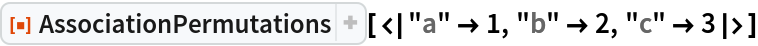Out=Get permutations of length two:

 In:=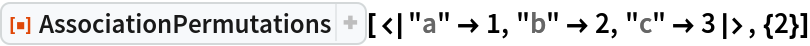Out=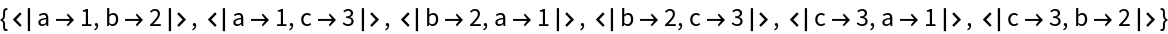### Scope (3)

Get permutations of all lengths, shortest ones first:

 In:=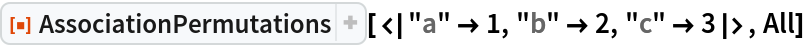Out=Lengths from one to two:

 In:=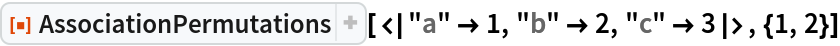Out=Lengths from one to three with step size two:

 In:=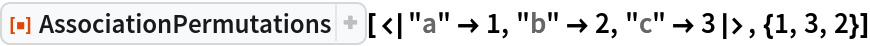Out=### Applications (2)

Kids love Yahtzee, but they always lose the dice. None of these kids have the requisite six dice to play:

 In:=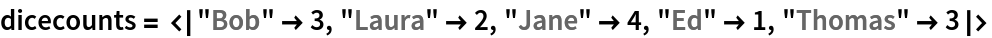Out=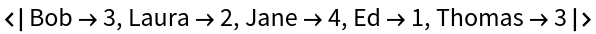Luckily, they can play together. Find the groups of kids that have at least six dice:

 In:=Out=### Properties and Relations (2)

Permutations does not support Association:

 In:=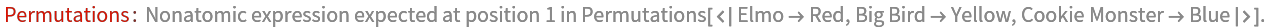Out=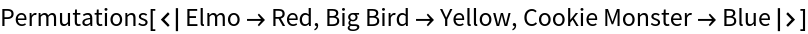In:=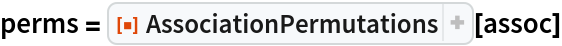Out=The result is equivalent to using Permutations on the Keys:

 In:=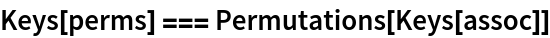Out=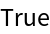AssociationPermutations does not evaluate delayed values:

 In:=Out=## Version History

• 1.0.0 – 30 September 2020